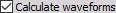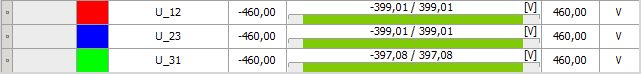# 3-phase outputs

To display all channels go to second tab (Setup) in edit module section and select the Channel list from the drop down menu.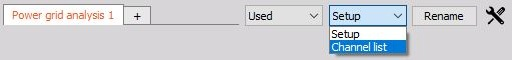NOTE: Following description and examples are valid for all types of 3 phase systems: 3-phase star, 3-phase Aron, 3-phase delta and 3-phase V.

Dewesoft automatically creates following Basic output channels

Current channels choice = disabled

Calculate line voltages choice = disabled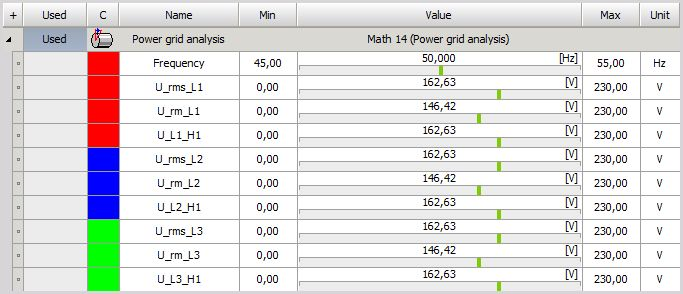To ‘Used’ analog channels voltage (U_Ln_H1 and U_Ln_rms) and frequency channels are added.   Current channels choice = disabled

Calculate line voltages choice = enabled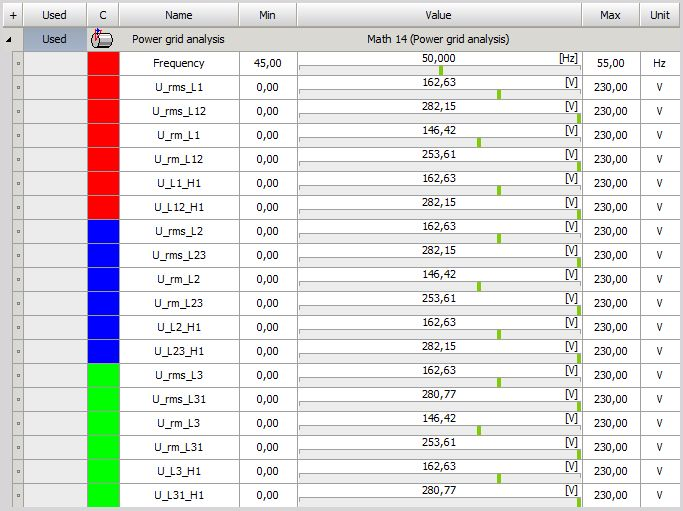To basic channels (see on left) emphasized channels are added -> between lines voltages channels.

Current channels choice = enabled

Calculate line voltages choice = disabled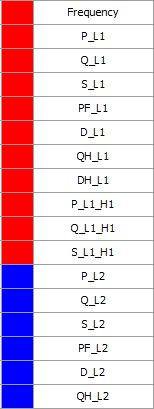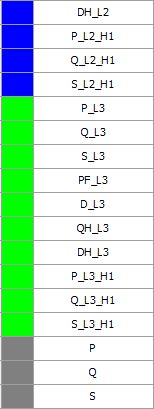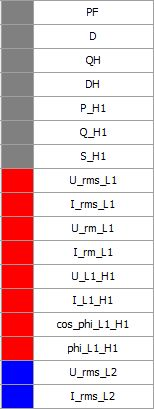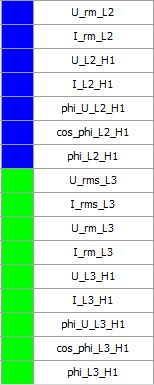To basic channels current and power channels are added.

When you compare channels on above picture with created channels for Single phase outputs, you can see that for 3 Phase systems only the same kind channels for second - L2 and 3rd - L3 phase line are added.

NOTE: H1 harmonics channels are created anyway for: voltage and current channels (U and I), power channels (P, Q, S, phi, cos phi).

### Harmonics output channels -> Harmonics option is checked

NOTE: Additional to Single phase Harmonics channels only the same kind output channels for second - L2 and 3rd - L3 phase line are created.

### THD output channels -> THD option is checked

NOTE: Additional to Single phase THD channels only the same kind output channels for second - L2 and 3rd - L3phase line are created.

### Symmetrical components -> Symmetrical components option is checked

We can calculate Symmetrical components by selecting the following check box.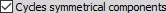Symetrical components are available only on three phase systems and tell us the unbalance of the grid.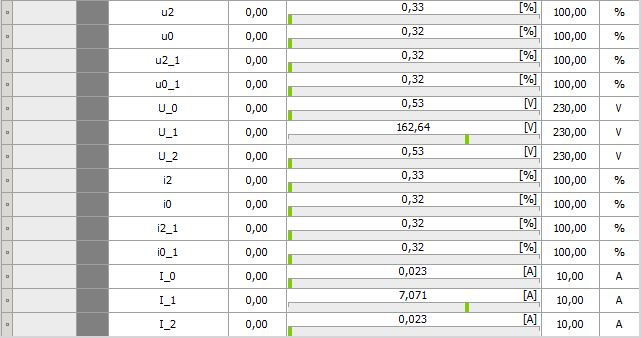### Period values output channels -> Period values option is checked

NOTE: Additional to Single phase Period values channels only the same kind output channels for second - L2 and 3rd - L3 phase line are created.

### Waveforms -> Waveforms option is checked

If we measure line to ground voltages, we can calculate Waveforms for the line to line voltages by selecting the following option: